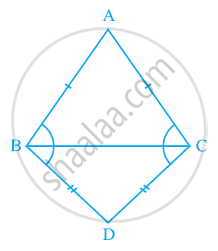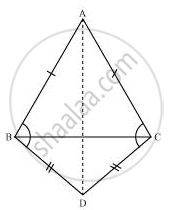# ABC and DBC are two isosceles triangles on the same base BC (see the given figure). Show that ∠ABD = ∠ACD. - Mathematics

ABC and DBC are two isosceles triangles on the same base BC (see the given figure). Show that ∠ABD = ∠ACD.#### SolutionIn ΔABD and ΔACD,

AB = AC (Given)

BD = CD (Given)

∴ ΔABD ~=ΔACD (By SSS congruence rule)

⇒ ∠ABD = ∠ACD (By CPCT)

Concept: Properties of a Triangle
Is there an error in this question or solution?

#### APPEARS IN

NCERT Class 9 Maths
Chapter 7 Triangles
Exercise 7.2 | Q 5 | Page 124